Browse by Topic
Related Topics

# New in the Wolfram Language: RandomPoints

Picking random points on the surface of a sphere so that the points are uniformly distributed is not as straightforward as you might think. Naively picking random spherical coordinates ϕ and θ will not give a uniform distribution of points. The problem is important enough to warrant a dedicated article in encyclopedias, such as Wolfram MathWorld (see Sphere Point Picking). Uniform sampling from Sphere[] is now available in the Wolfram Language with the RandomPoint function: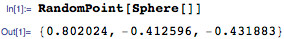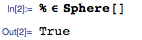In fact, RandomPoint can be used to uniformly sample from any bounded geometric region, in any dimension. In 2D: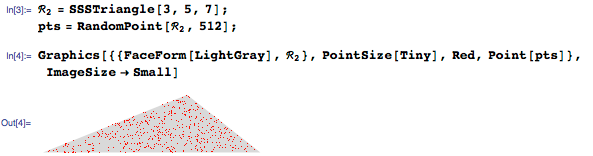In 3D: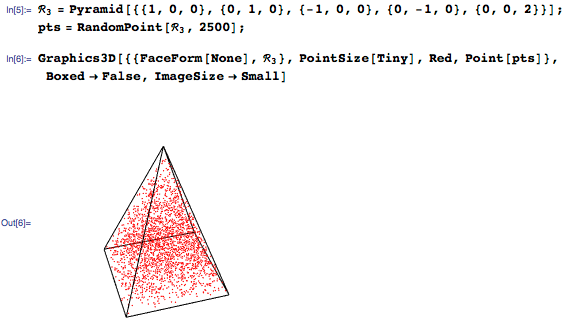In 10D: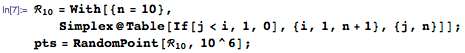Use the 10D points to estimate the region centroid: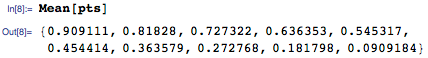Compare to the numerical value of the exact coordinates: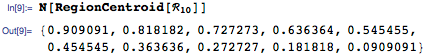RandomPoint aims to enable sampling from all the geometric regions supported in the Wolfram Language, including basic geometric regions, mesh regions, and formula and derived regions.

For example, you can use RandomPoint to mark uniformly distributed locations on a map of Africa: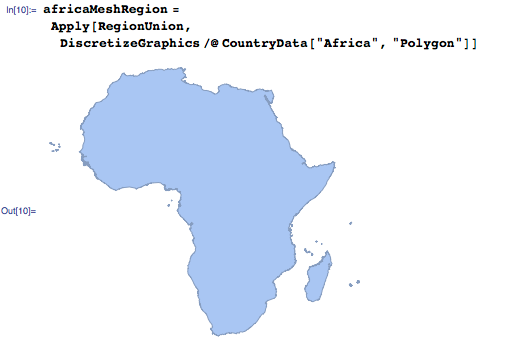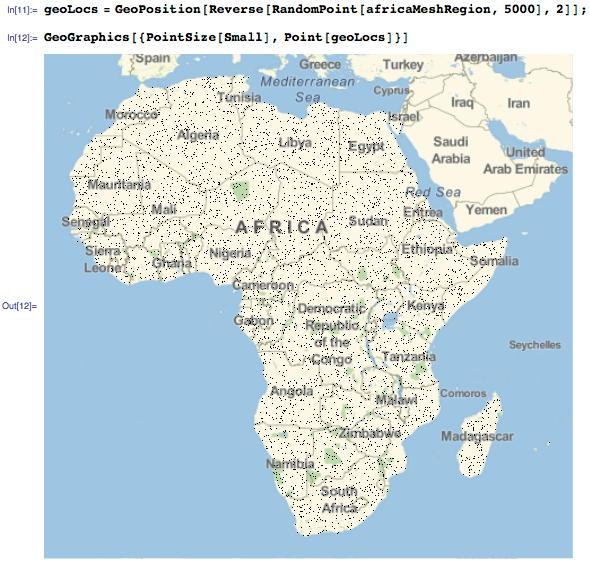Random points can be used to approximate geometric quantities. For instance, to estimate the maximum distance between two points in a regular pentagon inscribed in the unit circle, find the maximum distance between 1,000 pairs of random points on the boundary of a pentagon: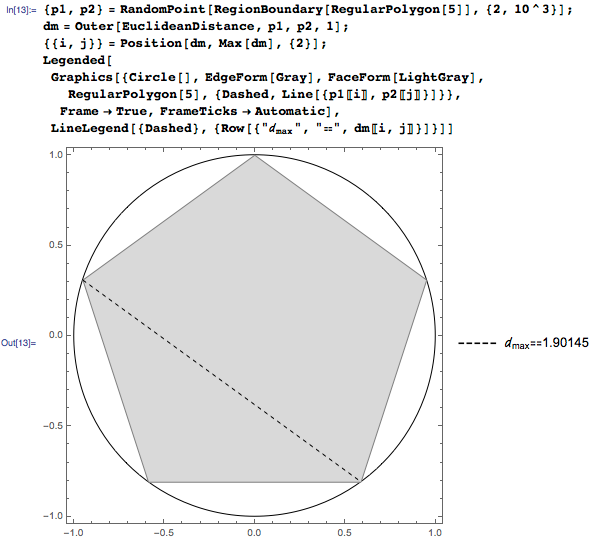Or estimate the area of the symmetric difference using RegionSymmetricDistance (or any Boolean combination) of region sets: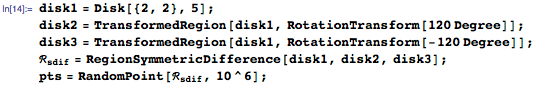Visualize the point cloud: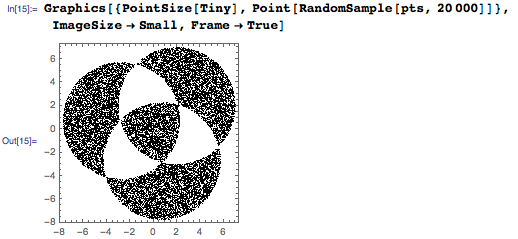Build the Nearest function to quickly test if a point is within a given distance r from the point cloud: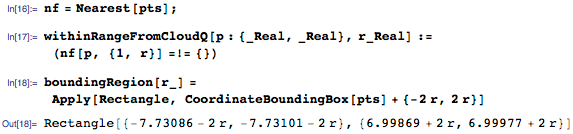Use the Monte Carlo method to estimate the area of the underlying region ℛ* from the set of sample points pts. This is done by sampling n points from the bounding rectangular region and counting the fraction of points that are within the range of r from the point cloud.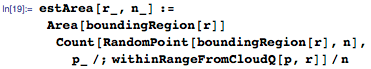Accumulate the estimate statistics: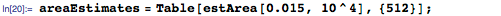Estimate the distribution density: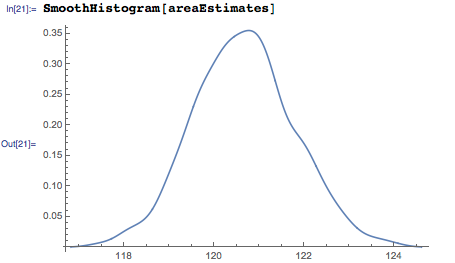Compare the estimated value with the exact numerical value: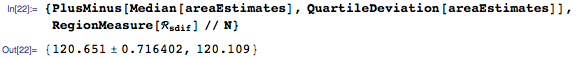I will conclude with a one-liner, a RandomPoint-based, Styrofoam-style visualization of the 8₃ knot: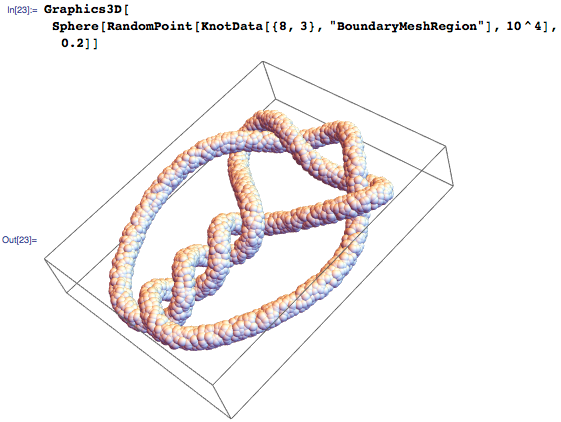RandomPoint is part of both the geometric computation and the probability and statistics capabilities in the Wolfram Language. RandomPoint was first introduced in Version 10.2 of the Wolfram Language and has been extended to cover new methods with the release of 10.3.